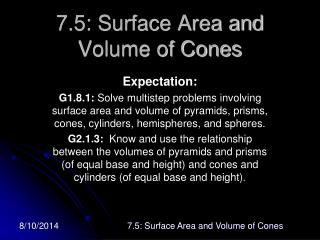# 7.5: Surface Area and Volume of Cones - PowerPoint PPT PresentationDownload Presentation7.5: Surface Area and Volume of Cones

7.5: Surface Area and Volume of ConesDownload Presentation## 7.5: Surface Area and Volume of Cones

- - - - - - - - - - - - - - - - - - - - - - - - - - - E N D - - - - - - - - - - - - - - - - - - - - - - - - - - -
##### Presentation Transcript

1. 7.5: Surface Area and Volume of Cones Expectation: G1.8.1: Solve multistep problems involving surface area and volume of pyramids, prisms, cones, cylinders, hemispheres, and spheres. G2.1.3: Know and use the relationship between the volumes of pyramids and prisms (of equal base and height) and cones and cylinders (of equal base and height). 7.5: Surface Area and Volume of Cones

2. Cone • Defn: A cone is a 3 dimensional figure that is the union of a circular region (base) and a curved lateral surface that connects the base to a single point (vertex) not in the plane of the base. 7.5: Surface Area and Volume of Cones

3. Altitude of a Cone The altitude of a cone is the segment with one endpoint at the vertex and the other endpoint in the plane of the base such that it is perpendicular to the base. 7.5: Surface Area and Volume of Cones

4. Height of a Cone • The height of a cone is the length of its altitude. 7.5: Surface Area and Volume of Cones

5. Axis of a Cone • The axis of a cone is a segment whose endpoints are the center of the base and the vertex of the cone 7.5: Surface Area and Volume of Cones

6. Right Cone • A cone is a ______ cone if its axis is also the altitude. 7.5: Surface Area and Volume of Cones

7. Oblique Cone • A cone is oblique if its axis is _____ its altitude. 7.5: Surface Area and Volume of Cones

8. Slant Height of a Cone • A slant height is a segment whose endpoints are the vertex and a point on the circle of the base, such that the segment is ________________ to the base edge. 7.5: Surface Area and Volume of Cones

9. Slant Height l The length of a slant height is denoted l. 7.5: Surface Area and Volume of Cones

10. Surface area of a Right Cone • Let L be the lateral area and S be the surface area of a right cone with radius r and slant height l. • Then L = __________ and • S = _________________. 7.5: Surface Area and Volume of Cones

11. A right cone has lateral area of 84π. If the slant height is 12 cm, what is the exact surface area of the cone? 7.5: Surface Area and Volume of Cones

12. Volume of a Cone • Let V be the volume of a cone with radius r and height h. • Then V =___________. 7.5: Surface Area and Volume of Cones

13. A paper cup is in the shape of a cone. If the diameter of the cone is 7cm and the height is 10cm, what is the capacity (in mL) of the cup? 7.5: Surface Area and Volume of Cones

14. A right rectangular prism is 4 inches long, 2 inches wide and 2.5 inches high. A right cone is 3 inches tall and its base has a radius of 2.5 inches. If the prisms was made of ice and it melted, would the cone be able to contain all of the water from the prism? 7.5: Surface Area and Volume of Cones

15. What is the volume of a cylinder with a height of 16 and a base area of 25π? • 5π • 25π • 50π • 200π • 400π 7.5: Surface Area and Volume of Cones

16. Assignment • pages 465-467, • #11-35 (odds), 32 7.5: Surface Area and Volume of Cones